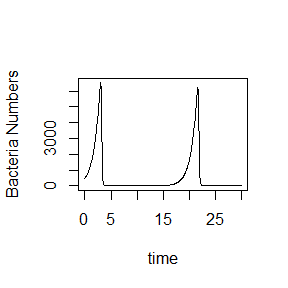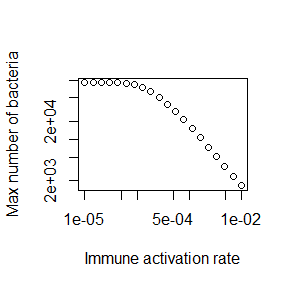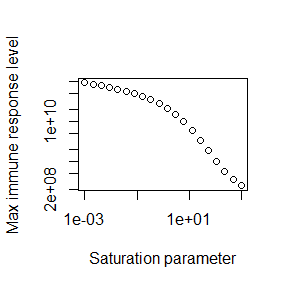# DSAIRM Package Tutorial

## Summary

Dynamical Systems Approaches to Immune Response Modeling (DSAIRM) is an R package that allows individuals to explore and learn about dynamical systems modeling of within-host infection and immune response dynamics, by interacting with models through a graphical user interface. It is not necessary - though possible - to read or write computer code.

The idea behind the specific structure of the package is that it provides a gentle introduction to immune response modeling that starts with a graphical user interface and takes students slowly to more advanced levels.

The different use cases for the package are described below.

## Package description

The package consists of several simulations/apps that allow for the simulation and exploration of different topics in within-host infection and immune response dynamics.

The underlying models are written as compartmental dynamical models, either deterministic using differential equations (deSolve package) or stochastic using a Gillespie-type approach (adaptivetau package). A graphical user interface is wrapped around each simulation/app. The graphical user interfaces are written using the functionality of the R Shiny package. This allows exploration of models without the need to write any code. At the same time, the package is structured in a modular way that should allow those interested in the actual models and learning R coding to easily move from one stage to another.

Each app is meant to be fully self-explanatory and contains a description of the model, a list of tasks the user could try, and information on further details and readings.

## Intended audience and goal of the package

The main audience are individuals who want to learn how mechanistic, dynamical systems models can be used to study within-host infection dynamics. While the ideal use of this package is likely as component of a class on this topic, self-learning might also be possible. Each app contains a fair amount of documentation and description of the model. By reading the model description, doing the tasks and probably also reading some of the papers in the future information section, it might be possible to teach yourself the beginnings of within-host modeling on your own.

## Installing the package

The package can be installed from CRAN or Github. See the documentation on the package page for more details.

Package installation is a one-time process, unless R itself is being upgraded/reinstalled. Note that the package depends on other packages, which will also be installed as needed.

## Using the package

The following sections describe the main envisioned ways the content in this R package can be used and extended. The idea is that everyone starts at level 1, and then, based on needs and interests, can decide to move on to the next level.

### Level 1: Interactive use through the graphical user interface

The interactive exploration of the models and infectious disease concepts through the graphical user interface is the main intended use of this package. The steps to get there are simple.

#### Load and run the package

Every time a new R/Rstudio session is started, the package needs to be loaded:

library('DSAIRM')

followed by starting the main menu for the package:

dsairmmenu()

This will bring up a graphical menu from which one can select each Shiny app. Each app contains the information needed to understand the underlying model, and has a list of (non exhaustive) suggested tasks to learn about the topic covered by the app. After exploring an app, the user returns to the main menu and eventually exits the main menu and closes the R session. No code needs to be written at this level of exploration and learning.

### Level 2: Directly interacting with the simulation functions

The exploration of the models through the graphical interface is the first and main intended use of the package. Once you are comfortable interacting with the models and have a good understanding of the concepts covered by the different apps, it is possible, without too much effort, to interact with the code more directly. This will provide more flexibility but will require writing some code.

To facilitate direct interaction and modification of the underlying simulations, each app is structured in such a way that the underlying model/simulation is a stand-alone function. For some apps, there are multiple underlying functions involved. You can call/use any of these functions directly, without going through the graphical interface. The ‘Further Information’ tab inside each app provides the name of the corresponding underlying function(s).

Consider as example the first app, called Basic bacteria model. This model has 2 underlying simulator functions, one that runs the discrete-time model called simulate_basicbacteria_discrete.R and one that runs the continuous, differential equation model called simulate_basicbacteria_ode.R. If you were interested in the latter, you can learn about the inputs and outputs of the function by looking at its documentation. To that end, type the following into the R console (make sure you loaded the DSAIRM package first):

help('simulate_basicbacteria_ode')

The help file explains that one can run the simulation by specifying initial number of bacteria and immune response strength, the duration for which the simulation should be run, and the different model parameters. Unless explicitly specified, the models do not have inherent time units. Instead, those are set by the user based on choices for parameters. Each parameter has some default, which is used if one calls the fuction without any additional information. You can modify those default settings. For instance you could call the simulator with the following settings, overwriting the defaults for some of the parameters, while using the default values for the remainder:

result <- simulate_basicbacteria_ode(B = 500, I = 5, g = 1, r = 0.002, dI = 1 )

Calling the simulation function executes the underlying dynamical model. The simulation function produces and returns time-series for the dynamics of each of the variables that are tracked. It is now your job to produce their own plots. As example, a plot of bacteria as function of time could be achieved with this line of code:

plot(result$ts[,"time"],result$ts[,"B"],xlab='time',ylab='Bacteria Numbers',type='l')The ability to call the simulation functions directly instead of going through the graphical interface allows additional exploration of the models. For instance if one wanted to explore the behavior of a model systematically for different values of a given parameter, this would need to be done manually if run through the graphical interface. Calling the function directly allows one to automate this by wrapping the function inside a loop over the parameter of interest, recording some quantity of interest for each run, and report the result at the end. The following is a simple example, showing a loop over different values of the immune response activation rate and recording the peak of the bacteria numbers each time, with the final result peak of bacteria as function of immune activation rate shown in a plot:

rvec = 10^seq(-5,-2,length=20) #values of log immune activation rate, r, for which to run the simulation
peak = rep(0,length(rvec)) #this will record the peak values for each r
for (n in 1:length(rvec))
{
#call the simulator function with different values of r, other parameters stay at their defaults
result <- simulate_basicbacteria_ode(r = rvec[n])
peak[n] <- max(result$ts[,"B"]) #record max number of bacteria for each value of r } #plot final result plot(rvec,peak,type='p',xlab='Immune activation rate',ylab='Max number of bacteria',log='xy')Thus, you can add your own custom code to the existing simulator functions and with a few lines of extra code analyze and explore many more questions and scenarios than those accessible through the graphical interface. This provides a lot more flexibility, but requires writing some R code to interface with the supplied simulator functions. ### Level 3: Modifying the simulation functions Level 2 provides a user with some flexibility, but the main constraint is that only existing DSAIRM models can be used and analyzed. This constraint can be overcome by directly modifiying and customizing the underlying simulation functions. As was true for moving from level 1 to 2, this move from level 2 to what I call level 3 provides further (almost unlimited) flexibility at the cost of having to write increasingly more R code. To make modifying the existing functions easy, copies of all simulator functions are provided in a subdirectory called simulatorfunctions inside the DSAIRM package folder. Each function in that folder starts with simulate_. The following R command will point the user to the location of this directory on their computer: system.file("simulatorfunctions", package = "DSAIRM") Don’t edit the functions in this subfolder (they are being used by the package as well), but instead to copy the whole folder to a new location and then work with the copies of these functions. The code for each simulator function is (hopefully) well documented. Some basic to intermediate level of R coding experience is likely required to successfully modify the functions. In addition to modifying the simulator function of interest, a user will likely also have to write some additional code to interact with their modified function (as described in Level 2). The following provides an illustrative example of this approach. Assume that a user wants to modify the simple bacteria model ODE model. The code for this model is in simulate_basicbacteria_ode.R. (All functions are in files with the corresponding name.) After finding the file, making a copy and renaming it (called mysimulator.R here), the user can make modifications. Let’s say the user wants to study a specific system for which this simple model is suitable. However in their system, it is biologically more reasonable to assume that the immune system growth term includes saturation at some maximum rate when bacteria numbers are high. This can be accomplished by a change of the term rBI to rBI/(B+s). (See the documentation for this app for an explanation of each model term and corresponding equation). This change leads to a growth at rate rB proportional to the number of bacteria if B is smaller than some threshold parameter s, and turns into a growth at fixed maximum rate r, independent of bacteria numbers, if B is larger than s. The following modifications to the code will accomplish this: old: simulate_basicbacteria_ode <- function(B = 10, I = 1, g = 1, Bmax = 1e+05, dB = 0.1, k = 1e-06, r = 0.001, dI = 1, tstart = 0, tfinal = 30, dt = 0.05) new: mysimulator <- function(B = 10, I = 1, g = 1, Bmax = 1e+05, dB = 0.1, k = 1e-06, r=1e3, dI=1, tstart = 0, tfinal = 30, dt = 0.05, s=1E3) Note the changed default value for r to ensure the immune response is sufficiently triggered. old: pars = c(g=g,Bmax=Bmax,dB=dB,k=k,r=r,dI=dI) new: pars = c(g=g,Bmax=Bmax,dB=dB,k=k,r=r,dI=dI,s=s) old: dBdt = g*B*(1-B/Bmax) - dB*B - k*B*I dIdt = r*B*I - dI*I new: dBdt = g*B*(1-B/Bmax) - dB*B - k*B*I dIdt = r*B*I/(s+B) - dI*I Note that if we wanted to share this function with others, we would need to also update the function documentation at the start of the file. For personal use of the function, this is a good idea but not required. With these changes made, it is now possible to use the new mysimulator function to ask specific questions regarding this model (and thus, it’s underlying biological system.) For instance one can explore how different values of the saturation parameter s impact the maximum level of the immune response. This requires a slight modification of the code shown above in Level 2 as follows: source('mysimulator.R') #to initialize the new function - it needs to be in the working directory svec = 10^seq(-3,3,length=20) #values of saturation parameter Imax = rep(0,length(svec)) #this will record the maximum immune response level for (n in 1:length(svec)) { result <- mysimulator(s = svec[n]) #all other inputs are kept at their defaults Imax[n] <- max(result$ts[,"I"])
}
plot(svec,Imax,type='p',xlab='Saturation parameter',ylab='Max immune response level',log='xy')For most users and especially novice coders, it is likely best to start with one of the provided models and modify as needed. However, at this level, a user has essentially full control and is only limited by what can be accomplished using the R programming language and their ability and interest in writing customized code.

## Contributing to the package

The package is on GitHub and you can use the usual GitHub process to contribute updated, bug fixes, etc. If you don’t know how to do that or don’t have the time, you can also file an issue on GitHub and let me know what should be changed.

The package is built in a way that makes it (hopefully) easy for others to contribute new simulations/apps. To that end, the package contains this Markdown file, documentation.md, which provides further information on the details of the package structure. If you plan to develop new apps, or add other substantial updates, it’s best to get in touch with me first via email or GitHub.

## Further information

• The package vignette provides details about the different ways the package can be used. I highly recommend going through it.
• The documentation.md file described above contains more information about the package structure.
• For feedback, bug reports, feature requests, etc., file a GitHub issue.
• A ‘companion’ package to this one, called Dynamical Systems Approaches for Infectious Disease Epidemiology (DSAIDE), focuses on models for infectious disease epidemiology (the population level). It has the same structure as DSAIRM. See the DSAIDE site for more information.